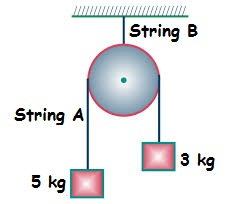Mechanics - 1Two blocks of mass $\text{5 kg}$ and $\text{3 kg}$ are suspended from a pulley with string $A$. The pulley is hung from ceiling through another string $B$. The tension in strings $A$ and $B$ are $T_A$ and $T_B$ newtons respectively. The blocks move with an acceleration $a \text{ m/s}^2$.

Find the sum of the magnitudes of $a$, $T_A$, and $T_B$.

Assumptions and Details:

• Both strings are massless and inextensible.
• The pulley is massless and frictionless between the strings and pulley.
• Acceleration due to gravity $g = \text{10 m/s}^2$.

Try my World of Physics to solve many problems like this one.

×